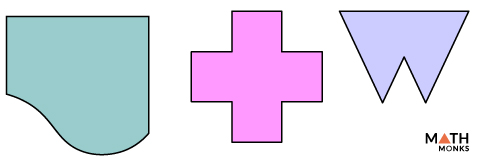# Line of Symmetry

The line of symmetry is an imaginary line that divides a shape or an object into identical halves. It is also called the axis of symmetry or the mirror line since it divides the figure symmetrically.

The diagram below shows how a line of symmetry divides a shape into identical halves that are mirror images.

## Types

Based on the orientation of the symmetry line of an object, lines of symmetry are of 3 types:

### Vertical Line of Symmetry

It is an imaginary vertical line that goes from top to bottom (or vice-versa) in an object and divides it into right and left mirror halves. For example, a club shape has a vertical line of symmetry.

### Horizontal Line of Symmetry

It is an imaginary horizontal line that goes from right to left (or vice-versa) in an object and divides it into top and bottom mirror halves. For example, an arrow has a horizontal line of symmetry.

### Diagonal Line of Symmetry

It is an imaginary diagonal or skew line that goes slanting in an object and divides it into mirror halves, as in the case of a square.

## Number

An object can have one or more lines of symmetry. Some examples involving real-life objects are:

In geometry, the number of lines of symmetry varies according to the shape as shown in case of polygons.

• In triangles, the line of symmetry can be one, three, or zero, depending upon its type.
• In quadrilaterals, the line of symmetry can be one, two, four, or zero based on the shape.
• In regular polygons, the line of symmetry can be multiple depending on the number of sides.
• A circle has infinite lines of symmetry as an infinite number of lines pass through its center.

## Solved ExamplesFind the number of lines of symmetry in the shapes given along side.

Solution:The first shape is asymmetric. So it has no lines of symmetry.
The second shape has 4 lines of symmetry.
The third shape has only 1 line of symmetry.

## FAQs

Q1. How many lines of symmetry does a rhombus have?

Ans. A rhombus has 2 lines of symmetry. They are the diagonals.

Q2. How many lines of symmetry does the isosceles trapezoid have?

Ans. An isosceles trapezoid has 1 line of symmetry, which is the vertical line of symmetry.

Q3. How many lines of symmetry does an equilateral triangle have?

Ans. An equilateral triangle has 3 lines of symmetry.

Q5. How many lines of symmetry does a parallelogram have?

Ans. A parallelogram has no line of symmetry.

Q6. How many lines of symmetry does a circle have?

Ans. A circle has infinite number of symmetry.